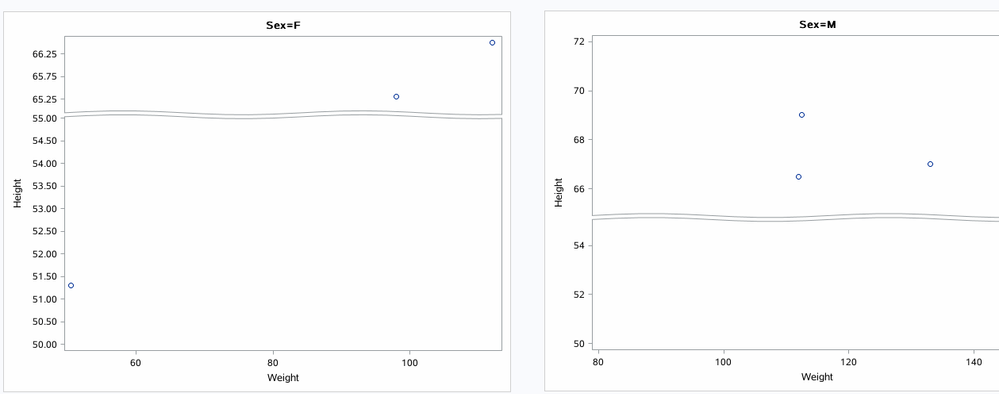## Create Broken line y-axis when using a datapanel layout

Dear community,

Creating a broken y-axis with a range of value can be accomplished using the lattice layout.

Does anyone tried to create the same broken y-axis across different panels when the datapanel layout is

used?

Regards

Santos

2 REPLIES 2Ksharp
Super User

## Re: Create Broken line y-axis when using a datapanel layout

Can you use PROC SGPLOT ? like:``````proc sort data=sashelp.class out=class;
by sex;
run;
ods layout gridded advance=bygroup columns=2 ;
proc sgplot data=class;
by sex;
scatter x=weight y=height;
yaxis ranges=(50-55 65-max) ;
run;
ods layout end;``````

## Re: Create Broken line y-axis when using a datapanel layout

Thank you for the answer. I did try in a previous iteration this solution.

It could work if I want to respect the proportion related to the y-axis range but  90% of the data are in the range 0-300 with some outliers in the range 1000-1500. Therefore, I want to expand the visibility in the range 0-300 (ie 90% of the final figure) and reduce/compress the apparent proportion for the outliers (ie 10%) (see attached figure using GTL) and the following code.

proc template;
define statgraph brokenlineplot;
begingraph;

/* Here we are reporting scattergraph */
layout lattice / rows = 2 rowweights=(.2 .8) rowdatarange=union rowgutter=2;

/* 1st row with "outliers" reported in this area with (xvar,yvar2) values */
/* You have to define new ymin (ie ymin1) and new ymax (ie ymax1) for this row with new list of tick
values (ie step1) */

layout overlay /
xaxisopts = (display= none linearopts=(viewmin = &xmin. viewmax=&xmax.)) /*  No values for x-Axis should be displayed */
yaxisopts= (display= (ticks tickvalues) linearopts=(
viewmin = &ymin1.
viewmax = &ymax1.
tickvaluesequence=(start= &ymin1. end = &ymax1. increment = &step1.)
)
);

scatterplot  x = &xvar.   y = yvar2;
%if %length(&xrefline.) %then %do;
/*I added this reference line which is the only one cutting the 1st row of the lattice */
referenceline x = &xrefline. / lineattrs=(color=grey pattern=dash) ;
%end;
endlayout;

/* 2nd row without outliers reported in this area with (xvar,yvar2) values */
/* You have to determine ymin and  ymax for this row with new list of tick
values */

layout overlay /
xaxisopts=(display= all  label = "&xlabel." /* offsetmin=0*/  linearopts=(
viewmin = &xmin. viewmax=&xmax.
tickvaluesequence=(start= &xmin. end = &xmax. increment = &xstep.)

)
)
yaxisopts= (display=all  label= "&ylabel." linearopts=(
viewmin = &ymin.
viewmax = &ymax.
tickvaluesequence=(start= &ymin. end = &ymax. increment = &step.)
)
);

scatterplot  x = &xvar. y = &yvar.  ;

%if %length(&yrefline.) %then %do;
referenceline y=&yrefline. / lineattrs=(color=grey pattern=dash);
%end;
/*I added this line to have both reference line at x = 0 */
%if %length(&xrefline.) %then %do;
referenceline x=&xrefline. / lineattrs=(color=grey pattern=dash);
%end;;
endlayout;
endlayout;

/*%end;*/
endgraph;
end;
run;

proc sgrender data= BrokenLine  template=brokenlineplot;
run;
quit;

Where the ranges for each row are dynamically determined

Discussion stats
• 2 replies
• 334 views
• 0 likes
• 2 in conversation